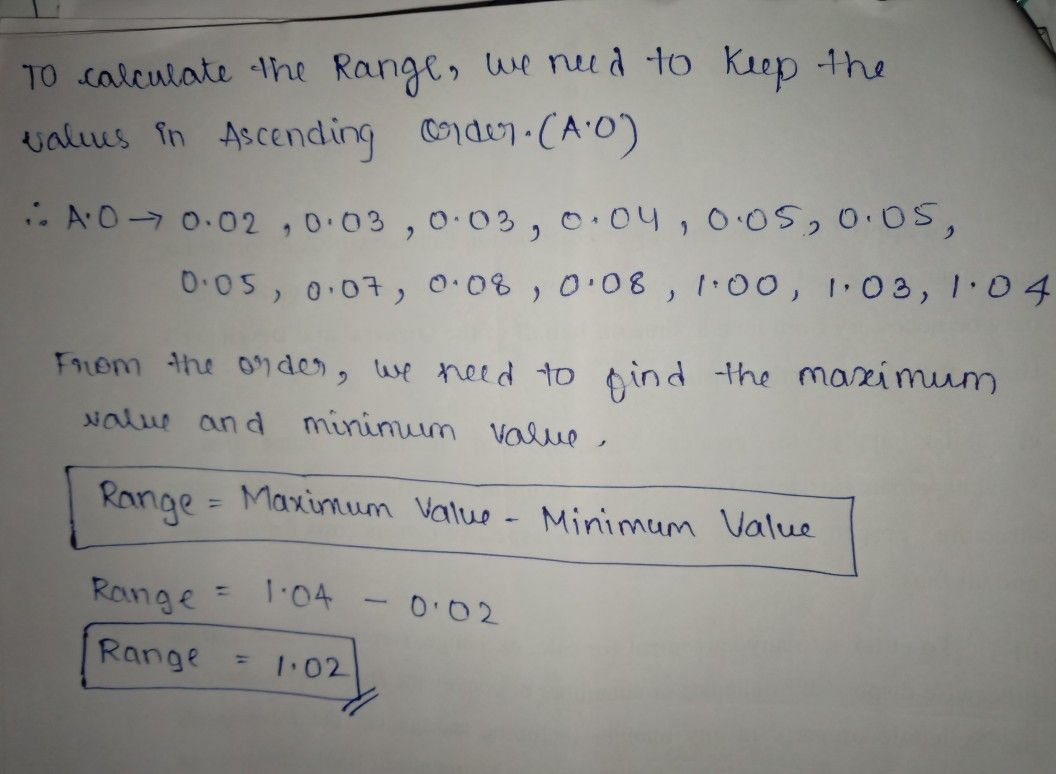Symbol
Problem$10$ 10.Calculate the range of the $data$ $0.03,$ $0.05,$ $1.04,$ $0.08,$ $0.05,1.03,$ $0.03,$ $0.04,0.07,0.05$ $0.02,1.00,$ $0.08.$
7th-9th grade
Probability and Statistics
Search count: 106
SolutionQanda teacher - KavithaIf you have any doubts please do let me know dear. if you are satisfied with my answer please do LIKE and give a 5 STAR RATING and coins as THANK U GIFT..! ALL THE BEST..!!!
Hope u understood dear